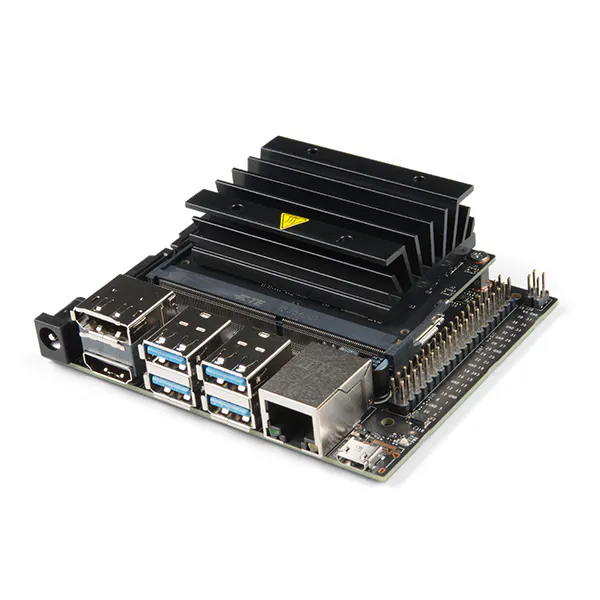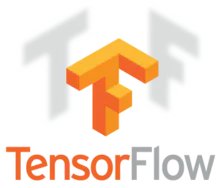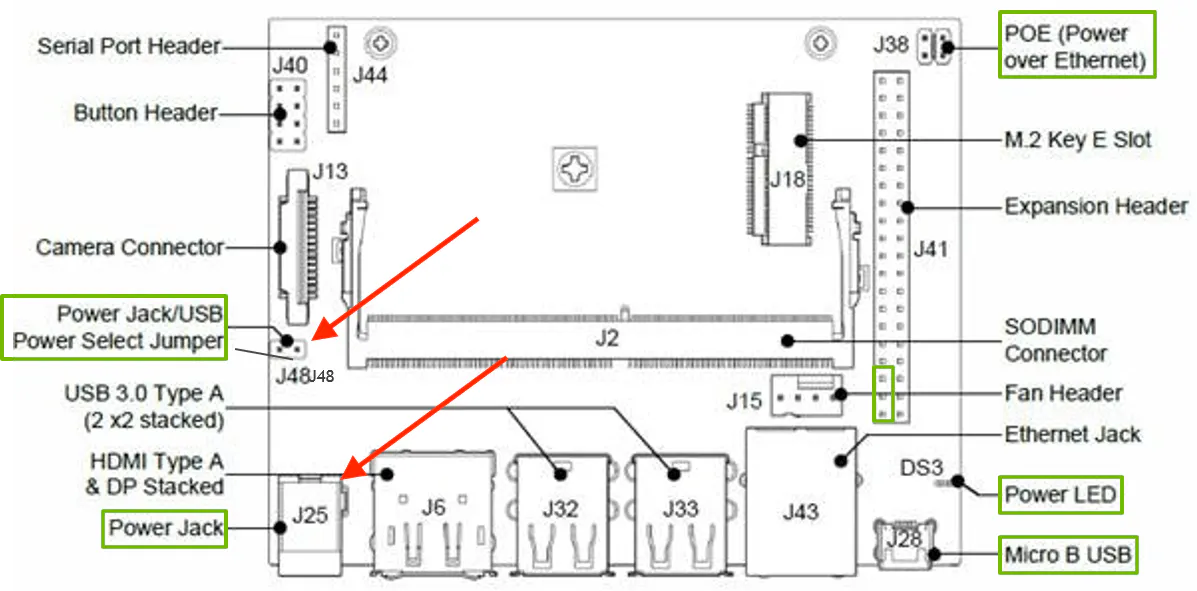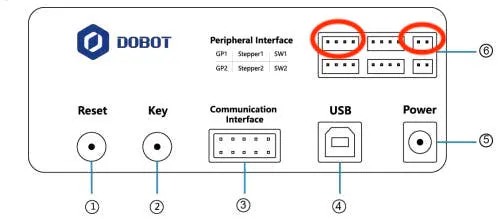Created November 15, 2019 © GPL3+

# AI Trash and Recyclable Sorting Robot Arm

Using AI on the edge to classify different types of recycles robotic arm for sorting.

## Things used in this project

### Hardware componentsNVIDIA Jetson Nano Developer Kit
×1
 Dobot Magician
×1

### Software apps and online services

 NVIDIA JetPack SDKTensorFlow
 labelImg

## Schematics

### Jetson Schematic

Jetson Schematic### Dobot ports

Dobot Suction cup ports## Code

### robot_ai_recycle.py

Python
The code for AI Robot Recycle sorting, leveraging tensorflow. Easiest way to execute this code is to drop it into object detection folder in tensorflow's mode/research/object_detection
```# Import packages
import os
import cv2
import numpy as np
import tensorflow as tf
import sys
import time
from serial.tools import list_ports
from pydobot import Dobot

# This is needed since the notebook is stored in the object_detection folder.
sys.path.append("..")

# Import utilites
from utils import label_map_util
from utils import visualization_utils as vis_util

# Name of the directory containing the object detection module we're using
MODEL_NAME = 'inference_graph'

# Grab path to current working directory
CWD_PATH = os.getcwd()

# Path to frozen detection graph .pb file, which contains the model that is used
# for object detection.
PATH_TO_CKPT = os.path.join(CWD_PATH,MODEL_NAME,'frozen_inference_graph.pb')

# Path to label map file
PATH_TO_LABELS = os.path.join(CWD_PATH,'training','labelmap.pbtxt')

# Number of classes the object detector can identify
NUM_CLASSES = 6

# Label maps map indices to category names, so that when our convolution
# network predicts `5`, we know that this corresponds to `king`.
# Here we use internal utility functions, but anything that returns a
# dictionary mapping integers to appropriate string labels would be fine
categories = label_map_util.convert_label_map_to_categories(label_map, max_num_classes=NUM_CLASSES, use_display_name=True)
category_index = label_map_util.create_category_index(categories)

# Load the Tensorflow model into memory.
detection_graph = tf.Graph()
with detection_graph.as_default():
od_graph_def = tf.GraphDef()
with tf.gfile.GFile(PATH_TO_CKPT, 'rb') as fid:
od_graph_def.ParseFromString(serialized_graph)
tf.import_graph_def(od_graph_def, name='')

sess = tf.Session(graph=detection_graph)

# Define input and output tensors (i.e. data) for the object detection classifier

# Input tensor is the image
image_tensor = detection_graph.get_tensor_by_name('image_tensor:0')

# Output tensors are the detection boxes, scores, and classes
# Each box represents a part of the image where a particular object was detected
detection_boxes = detection_graph.get_tensor_by_name('detection_boxes:0')

# Each score represents level of confidence for each of the objects.
# The score is shown on the result image, together with the class label.
detection_scores = detection_graph.get_tensor_by_name('detection_scores:0')
detection_classes = detection_graph.get_tensor_by_name('detection_classes:0')

# Number of objects detected
num_detections = detection_graph.get_tensor_by_name('num_detections:0')

# Initialize webcam feed
video = cv2.VideoCapture(0)
ret = video.set(3,1280)
ret = video.set(4,720)

# Init the robotic arm
port = list_ports.comports().device
device = Dobot(port=port, verbose=False)

# first move home
device.move_to(91, -88, 1, -47, wait=True)
isRoboticActive=False

# move left side
def _robotic_left():
global isRoboticActive
if isRoboticActive == False:
isRoboticActive = True
device.move_to(102, -94, 9, -135, wait=True)
device.move_to(185, -150, -58, -132, wait=True)
device.suck(True)
time.sleep(1)
device.move_to(214, 92, 5, -54, wait=True)
device.suck(False)
time.sleep(0.5)
device.move_to(193, -14, -13, -97, wait=True)
device.move_to(102, -94, 9, -135, wait=True)
isRoboticActive = False

# move right side
def _robotic_right():
global isRoboticActive
if isRoboticActive == False:
isRoboticActive = True
device.move_to(102, -94, 9, -135, wait=True)
device.move_to(185, -150, -58, -132, wait=True)
device.suck(enable=True)
time.sleep(1)
device.move_to(-75, -238, 34, -110, wait=True)
device.suck(enable=False)
time.sleep(0.5)
device.move_to(33, -191, -11, -173, wait=True)
device.move_to(102, -94, 9, -135, wait=True)
isRoboticActive = False

# function for robotic arm
def _move_robotic_arm(direction):
if direction == "left" and isRoboticActive == False:
elif direction == "right" and isRoboticActive == False:

while(True):

# Acquire frame and expand frame dimensions to have shape: [1, None, None, 3]
# i.e. a single-column array, where each item in the column has the pixel RGB value
frame_expanded = np.expand_dims(frame, axis=0)

# Perform the actual detection by running the model with the image as input
(boxes, scores, classes, num) = sess.run(
[detection_boxes, detection_scores, detection_classes, num_detections],
feed_dict={image_tensor: frame_expanded})

# Draw the results of the detection (aka 'visulaize the results')
vis_util.visualize_boxes_and_labels_on_image_array(
frame,
np.squeeze(boxes),
np.squeeze(classes).astype(np.int32),
np.squeeze(scores),
category_index,
use_normalized_coordinates=True,
line_thickness=8,
min_score_thresh=0.75)

#getting the detected item

i = 0
for x in np.squeeze(scores):
if x > .85:
print(np.squeeze(classes).astype(np.int32)[i])
result = np.squeeze(classes).astype(np.int32)[i]
#1 is cardboard
if result == 1:
_move_robotic_arm("left")
#3 is plastic
elif result == 3:
_move_robotic_arm("right")
break
i = i + 1

# All the results have been drawn on the frame, so it's time to display it.
cv2.imshow('AI Robotic Sorting', frame)

# Press 'q' to quit
if cv2.waitKey(1) == ord('q'):
break

# Clean up
device.close()
video.release()
cv2.destroyAllWindows()
```

### labelmap.pbtxt

Plain text
label for the categories
```item {
id: 1
name: 'Cardboard'
}

item {
id: 2
name: 'Glass'
}

item {
id: 3
name: 'Plastic'
}

item {
id: 4
name: 'Metal'
}

item {
id: 5
name: 'Trash'
}

item {
id: 6
name: 'Paper'
}
```

### ssdlite_mobilenet_v3_large_320x320_coco.config

Plain text
training config for ssdlite_mobilenet_v3_large_320x320_coco
```# SSDLite with Mobilenet v3 large feature extractor.
# Trained on COCO14, initialized from scratch.
# 3.22M parameters, 1.02B FLOPs
# TPU-compatible.
# Users should configure the fine_tune_checkpoint field in the train config as
# well as the label_map_path and input_path fields in the train_input_reader and
# eval_input_reader. Search for "PATH_TO_BE_CONFIGURED" to find the fields that
# should be configured.

model {
ssd {
inplace_batchnorm_update: true
freeze_batchnorm: false
num_classes: 6
box_coder {
faster_rcnn_box_coder {
y_scale: 10.0
x_scale: 10.0
height_scale: 5.0
width_scale: 5.0
}
}
matcher {
argmax_matcher {
matched_threshold: 0.5
unmatched_threshold: 0.5
ignore_thresholds: false
negatives_lower_than_unmatched: true
force_match_for_each_row: true
use_matmul_gather: true
}
}
similarity_calculator {
iou_similarity {
}
}
encode_background_as_zeros: true
anchor_generator {
ssd_anchor_generator {
num_layers: 6
min_scale: 0.2
max_scale: 0.95
aspect_ratios: 1.0
aspect_ratios: 2.0
aspect_ratios: 0.5
aspect_ratios: 3.0
aspect_ratios: 0.3333
}
}
image_resizer {
fixed_shape_resizer {
height: 320
width: 320
}
}
box_predictor {
convolutional_box_predictor {
min_depth: 0
max_depth: 0
num_layers_before_predictor: 0
use_dropout: false
dropout_keep_probability: 0.8
kernel_size: 39
use_depthwise: true
box_code_size: 4
apply_sigmoid_to_scores: false
class_prediction_bias_init: -4.6
conv_hyperparams {
activation: RELU_6,
regularizer {
l2_regularizer {
weight: 0.00004
}
}
initializer {
random_normal_initializer {
stddev: 0.03
mean: 0.0
}
}
batch_norm {
train: true,
scale: true,
center: true,
decay: 0.97,
epsilon: 0.001,
}
}
}
}
feature_extractor {
type: 'ssd_mobilenet_v3_large'
min_depth: 16
depth_multiplier: 1.0
use_depthwise: true
conv_hyperparams {
activation: RELU_6,
regularizer {
l2_regularizer {
weight: 0.00004
}
}
initializer {
truncated_normal_initializer {
stddev: 0.03
mean: 0.0
}
}
batch_norm {
train: true,
scale: true,
center: true,
decay: 0.97,
epsilon: 0.001,
}
}
override_base_feature_extractor_hyperparams: true
}
loss {
classification_loss {
weighted_sigmoid_focal {
alpha: 0.75,
gamma: 2.0
}
}
localization_loss {
weighted_smooth_l1 {
delta: 1.0
}
}
classification_weight: 1.0
localization_weight: 1.0
}
normalize_loss_by_num_matches: true
normalize_loc_loss_by_codesize: true
post_processing {
batch_non_max_suppression {
score_threshold: 1e-8
iou_threshold: 0.6
max_detections_per_class: 10
max_total_detections: 10
use_static_shapes: true
}
score_converter: SIGMOID
}
}
}

train_config: {
batch_size: 12
sync_replicas: true
startup_delay_steps: 0
replicas_to_aggregate: 32
num_steps: 400000
data_augmentation_options {
random_horizontal_flip {
}
}
data_augmentation_options {
ssd_random_crop {
}
}

fine_tune_checkpoint: "/home/ai/workspace/tensorflow1/models/research/object_detection/ssd_mobilenet_v3_large_coco_2019_08_14/model.ckpt"
fine_tune_checkpoint_type:  "detection"

optimizer {
momentum_optimizer: {
learning_rate: {
cosine_decay_learning_rate {
learning_rate_base: 0.4
total_steps: 400000
warmup_learning_rate: 0.13333
warmup_steps: 2000
}
}
momentum_optimizer_value: 0.9
}
use_moving_average: false
}
max_number_of_boxes: 10
}

input_path: "/home/ai/workspace/tensorflow1/models/research/object_detection/train.record"
}
label_map_path: "/home/ai/workspace/tensorflow1/models/research/object_detection/training/labelmap.pbtxt"
}

eval_config: {

}

input_path: "/home/ai/workspace/tensorflow1/models/research/object_detection/test.record"
}
label_map_path: "/home/ai/workspace/tensorflow1/models/research/object_detection/training/labelmap.pbtxt"
shuffle: false
}
```

### Recycle sorting robotic arm

Code base for Recycle Sorting Robotic Arm

## Credits

### Peter Ma

47 projects • 334 followers
Prototype Hacker, Hackathon Goer, World Traveler, Ecological balancer, integrationist, technologist, futurist.